# GMAT Math : DSQ: Understanding decimals

## Example Questions

### Example Question #1 : Decimals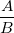is a fraction in lowest terms. Is its decimal representation a terminating decimal or a repeating decimal?

Statement 1:is a power of 5.

Statement 2:is a multiple of 7.

BOTH statements TOGETHER are sufficient to answer the question, but NEITHER statement ALONE is sufficient to answer the question.

BOTH statements TOGETHER are insufficient to answer the question.

Statement 1 ALONE is sufficient to answer the question, but Statement 2 ALONE is NOT sufficient to answer the question.

EITHER statement ALONE is sufficient to answer the question.

Statement 2 ALONE is sufficient to answer the question, but Statement 1 ALONE is NOT sufficient to answer the question.

Statement 2 ALONE is sufficient to answer the question, but Statement 1 ALONE is NOT sufficient to answer the question.

Explanation:

A fraction in lowest terms can be expressed as a terminating decimal if and only if its denominator has no prime factors other than 2 or 5; the numerator is irrelevant. Statement 1 is unhelpful since it only gives information about the numerator. If Statement 2 is assumed - that is, if we know the denominator has 7 as a prime factor - we know that the decimal representation ofis repeating.

### Example Question #2 : Decimalsandare fractions in lowest terms.

True or false: The result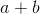can be represented by an integer or a terminating decimal.

Statement 1: Both fractions have powers of 5 in their denominators.

Statement 2: Both fractions have powers of 3 in their numerators.

BOTH statements TOGETHER are sufficient to answer the question, but NEITHER statement ALONE is sufficient to answer the question.

EITHER statement ALONE is sufficient to answer the question.

BOTH statements TOGETHER are insufficient to answer the question.

Statement 1 ALONE is sufficient to answer the question, but Statement 2 ALONE is NOT sufficient to answer the question.

Statement 2 ALONE is sufficient to answer the question, but Statement 1 ALONE is NOT sufficient to answer the question.

Statement 1 ALONE is sufficient to answer the question, but Statement 2 ALONE is NOT sufficient to answer the question.

Explanation:

Assume Statement 1 alone. A necessary and sufficient condition for a lowest-terms fraction to be equivalent to a terminating decimal is that its only prime factors are 2 and 5. Statement 1 says this about both fractions; the sum of terminating decimals is itself an integer or a terminating decimal.

Statement 2 alone provides insuffiicient information. Examine these examples: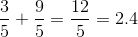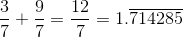Both fit the conditions of the main premise and Statement 2, but in only one case is the sum equal to a terminating decimal.

### Example Question #3 : Decimalsandare fractions in lowest terms.

True or false: The resultcan be represented by an integer or a terminating decimal.

Statement 1: Both denominators are even.

Statement 2: Both denominators are multiples of 3.

Statement 2 ALONE is sufficient to answer the question, but Statement 1 ALONE is NOT sufficient to answer the question.

Statement 1 ALONE is sufficient to answer the question, but Statement 2 ALONE is NOT sufficient to answer the question.

BOTH statements TOGETHER are sufficient to answer the question, but NEITHER statement ALONE is sufficient to answer the question.

BOTH statements TOGETHER are insufficient to answer the question.

EITHER statement ALONE is sufficient to answer the question.

BOTH statements TOGETHER are insufficient to answer the question.

Explanation:

Assume both statements to be true. A multiple of both 2 and 3 is also a multiple of 6, so both denominators are multiples of 6. Examine these two examples: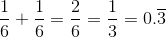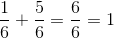In both examples, the conditions of the problem, including both statements, are met, but in only one is the sum equivalent to a terminating decimal or integer.

### Example Question #4 : Decimalsandare fractions in lowest terms.

True or false: The result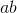can be represented by an integer or a terminating decimal.

Statement 1: Neither denominator is a multiple of 5.

Statement 2: Both denominators are powers of 2.

BOTH statements TOGETHER are insufficient to answer the question.

BOTH statements TOGETHER are sufficient to answer the question, but NEITHER statement ALONE is sufficient to answer the question.

Statement 2 ALONE is sufficient to answer the question, but Statement 1 ALONE is NOT sufficient to answer the question.

Statement 1 ALONE is sufficient to answer the question, but Statement 2 ALONE is NOT sufficient to answer the question.

EITHER statement ALONE is sufficient to answer the question.

Statement 2 ALONE is sufficient to answer the question, but Statement 1 ALONE is NOT sufficient to answer the question.

Explanation:

Assume Statement 1 alone. Examine these two examples: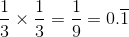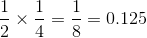Both fit the main condition and that of Statement 1, but in only one case is the sum equal to a terminating decimal or integer.

Assume Statement 2 alone. A necessary and sufficient condition for a lowest-terms fraction to be equivalent to a terminating decimal is that its only prime factors are 2 and 5. By Statement 2, both denominators have 2 as their only prime factor, so both can be represented by terminating decimals; their product must be terminating as well.

### Example Question #5 : Decimalsandare fractions in lowest terms.

True or false: The resultcan be represented by an integer or a terminating decimal.

Statement 1: The denominator ofis a power of 7.

Statement 2: The denominator ofis a power of 3.

EITHER statement ALONE is sufficient to answer the question.

Statement 1 ALONE is sufficient to answer the question, but Statement 2 ALONE is NOT sufficient to answer the question.

BOTH statements TOGETHER are insufficient to answer the question.

BOTH statements TOGETHER are sufficient to answer the question, but NEITHER statement ALONE is sufficient to answer the question.

Statement 2 ALONE is sufficient to answer the question, but Statement 1 ALONE is NOT sufficient to answer the question.

BOTH statements TOGETHER are insufficient to answer the question.

Explanation:

Assume both statements. Examine these two examples; both fit the main condition and those given in both statements.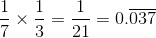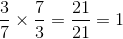In only one case is the product equivalent to an integer or a terminating decimal.

### Example Question #6 : Decimalsandare fractions in lowest terms.

True or false: The resultcan be represented by an integer or a terminating decimal.

Statement 1: The denominator ofis three times the numerator of.

Statement 2: The denominator ofis six times the numerator of.

Statement 1 ALONE is sufficient to answer the question, but Statement 2 ALONE is NOT sufficient to answer the question.

EITHER statement ALONE is sufficient to answer the question.

BOTH statements TOGETHER are sufficient to answer the question, but NEITHER statement ALONE is sufficient to answer the question.

Statement 2 ALONE is sufficient to answer the question, but Statement 1 ALONE is NOT sufficient to answer the question.

BOTH statements TOGETHER are insufficient to answer the question.

EITHER statement ALONE is sufficient to answer the question.

Explanation:

Assume Statement 1 alone. Rewrite the fractions as follows: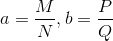, where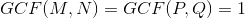- that is, their lowest-terms representations.

By Statement 1,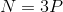, so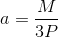. Therefore,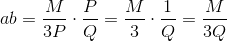Since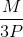is in lowest terms,is not a multiple of 3, so the 3 must remain in the denominator, even if theand the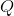can be reduced. Since a fraction which, in lowest terms, has a denominator with any prime factor other than 2 or 5 cannot be represented by an integer or a terminating decimal, Statement 1 proves thathas a repeating decimal as its equivalent.

A similar argument can be used to demonstrate that Statement 2 proves the same of.

### Example Question #7 : Decimalsandare fractions in lowest terms.

True or false: The resultcan be represented by an integer or a terminating decimal.

Statement 1: The denominator ofis five times the numerator of.

Statement 2: The denominator ofis eight times the numerator of.

BOTH statements TOGETHER are sufficient to answer the question, but NEITHER statement ALONE is sufficient to answer the question.

BOTH statements TOGETHER are insufficient to answer the question.

Statement 2 ALONE is sufficient to answer the question, but Statement 1 ALONE is NOT sufficient to answer the question.

EITHER statement ALONE is sufficient to answer the question.

Statement 1 ALONE is sufficient to answer the question, but Statement 2 ALONE is NOT sufficient to answer the question.

BOTH statements TOGETHER are sufficient to answer the question, but NEITHER statement ALONE is sufficient to answer the question.

Explanation:

Assume Statement 1 alone. Examine these examples: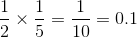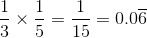In both cases, the main conditon and that of Statement 1 are satisfied, but in only one case can the result be represented by a terminating decimal or integer.

A similar argument shows Statement 2 alone to be insufficient: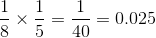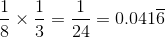Assume both statements. Then rewrite the fractions as follows:, where- that is, their lowest-terms representations.

By Statement 1,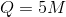; by Statement 2,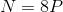. Therefore,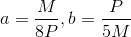,

and their product is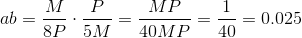,

a terminating decimal.

### Example Question #8 : Decimals

Solve forin the following equation.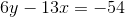I)is equivalent to seventy nine hundredths.

II) The slope of the line parallel to this equation is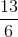.

Both statements are needed to answer the question.

Statement II is sufficient to answer the question, but statement I is not sufficient to answer the question.

Statement I is sufficient to answer the question, but statement II is not sufficient to answer the question.

Either statement is sufficient to answer the question.

Statement I is sufficient to answer the question, but statement II is not sufficient to answer the question.

Explanation:

We have one equation and two unknowns. To find x, we need to find y.

I) Tells us that y is .79 therefore we can plug this value into our equation and solve for x.Plugging in the y value from Statement I and using algebraic operations to solve for x.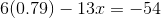Multiply first term.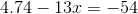Subtract 4.74 from both sides.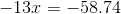Divide by -13.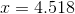II) Is irrelevant.

Therefore Statement I) alone is sufficient to answer the question but Statement II) alone is not.

Tired of practice problems?

Try live online GMAT prep today.